# DesignScript Geometry Basics

The simplest geometrical object in the Dynamo standard geometry library is a point. All geometry is created using special functions called constructors, which each return a new instance of that particular geometry type. In Dynamo, constructors begin with the name of the object’s type, in this case Point, followed by the method of construction. To create a three dimensional point specified by x, y, and z Cartesian coordinates, use the ByCoordinates constructor: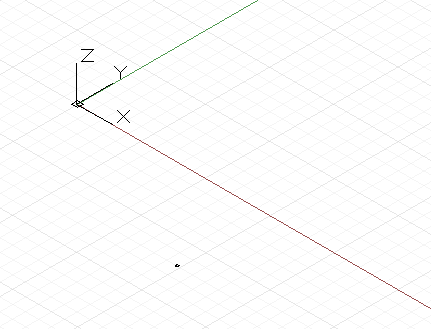``````// create a point with the following x, y, and z
// coordinates:
x = 10;
y = 2.5;
z = -6;

p = Point.ByCoordinates(x, y, z);
``````

Constructors in Dynamo are typically designated with the “By” prefix, and invoking these functions returns a newly created object of that type. This newly created object is stored in the variable named on the left side of the equal sign.

Most objects have many different constructors, and we can use the BySphericalCoordinates constructor to create a point lying on a sphere, specified by the sphere’s radius, a first rotation angle, and a second rotation angle (specified in degrees):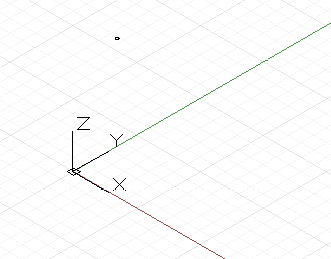``````// create a point on a sphere with the following radius,
// theta, and phi rotation angles (specified in degrees)
theta = 75.5;
phi = 120.3;
cs = CoordinateSystem.Identity();

phi);
``````

Points can be used to construct higher dimensional geometry such as lines. We can use the ByStartPointEndPoint constructor to create a Line object between two points: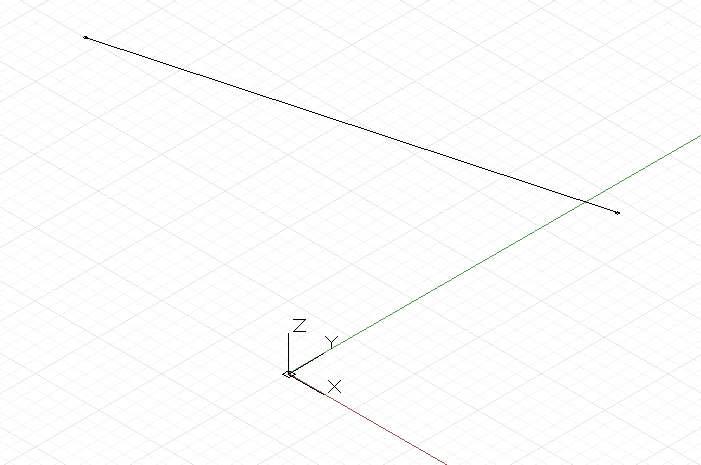``````// create two points:
p1 = Point.ByCoordinates(3, 10, 2);
p2 = Point.ByCoordinates(-15, 7, 0.5);

// construct a line between p1 and p2
l = Line.ByStartPointEndPoint(p1, p2);
``````

Similarly, lines can be used to create higher dimensional surface geometry, for instance using the Loft constructor, which takes a series of lines or curves and interpolates a surface between them.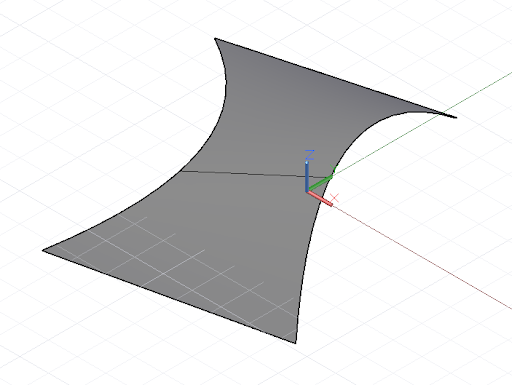``````// create points:
p1 = Point.ByCoordinates(3, 10, 2);
p2 = Point.ByCoordinates(-15, 7, 0.5);

p3 = Point.ByCoordinates(5, -3, 5);
p4 = Point.ByCoordinates(-5, -6, 2);

p5 = Point.ByCoordinates(9, -10, -2);
p6 = Point.ByCoordinates(-11, -12, -4);

// create lines:
l1 = Line.ByStartPointEndPoint(p1, p2);
l2 = Line.ByStartPointEndPoint(p3, p4);
l3 = Line.ByStartPointEndPoint(p5, p6);

// loft between cross section lines:
surf = Surface.ByLoft([l1, l2, l3]);
``````

Surfaces too can be used to create higher dimensional solid geometry, for instance by thickening the surface by a specified distance. Many objects have functions attached to them, called methods, allowing the programmer to perform commands on that particular object. Methods common to all pieces of geometry include Translate and Rotate, which respectively translate (move) and rotate the geometry by a specified amount. Surfaces have a Thicken method, which take a single input, a number specifying the new thickness of the surface.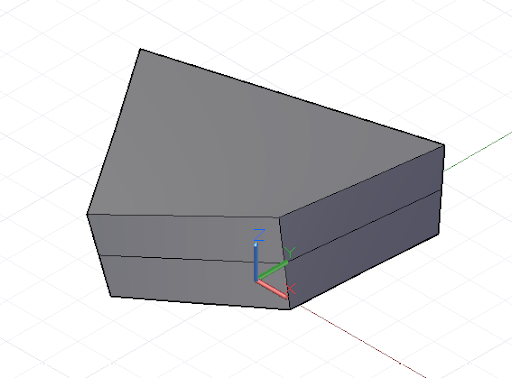``````p1 = Point.ByCoordinates(3, 10, 2);
p2 = Point.ByCoordinates(-15, 7, 0.5);

p3 = Point.ByCoordinates(5, -3, 5);
p4 = Point.ByCoordinates(-5, -6, 2);

l1 = Line.ByStartPointEndPoint(p1, p2);
l2 = Line.ByStartPointEndPoint(p3, p4);

surf = Surface.ByLoft([l1, l2]);

// true indicates to thicken both sides of the Surface:
solid = surf.Thicken(4.75, true);
``````

Intersection commands can extract lower dimensional geometry from higher dimensional objects. This extracted lower dimensional geometry can form the basis for higher dimensional geometry, in a cyclic process of geometrical creation, extraction, and recreation. In this example, we use the generated Solid to create a Surface, and use the Surface to create a Curve.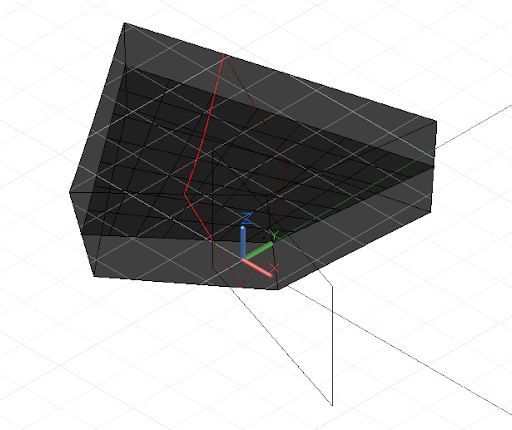``````p1 = Point.ByCoordinates(3, 10, 2);
p2 = Point.ByCoordinates(-15, 7, 0.5);

p3 = Point.ByCoordinates(5, -3, 5);
p4 = Point.ByCoordinates(-5, -6, 2);

l1 = Line.ByStartPointEndPoint(p1, p2);
l2 = Line.ByStartPointEndPoint(p3, p4);

surf = Surface.ByLoft([l1, l2]);

solid = surf.Thicken(4.75, true);

p = Plane.ByOriginNormal(Point.ByCoordinates(2, 0, 0),
Vector.ByCoordinates(1, 1, 1));

int_surf = solid.Intersect(p);

int_line = int_surf.Intersect(Plane.ByOriginNormal(
Point.ByCoordinates(0, 0, 0),
Vector.ByCoordinates(1, 0, 0)));
``````